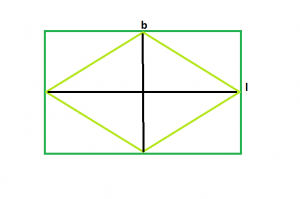# Area of the biggest possible rhombus that can be inscribed in a rectangle

Given a rectangle of length l and breadth b, the task is to find the largest rhombus that can be inscribed in the rectangle.

Examples:

```Input : l = 5, b = 4
Output : 10

Input : l = 16, b = 6
Output : 48
```

## Recommended: Please try your approach on {IDE} first, before moving on to the solution.From the figure, we can see, the biggest rhombus that could be inscribed within the rectangle will have its diagonals equal to the length & breadth of the rectangle.
So, Area of rhombus, A = (l*b)/2

Below is the implementation of the above approach:

## C++

 `// C++ Program to find the biggest rhombus ` `// which can be inscribed within the rectangle ` `#include ` `using` `namespace` `std; ` ` `  `// Function to find the area ` `// of the biggest rhombus ` `float` `rhombusarea(``float` `l, ``float` `b) ` `{ ` `    ``// the length and breadth cannot be negative ` `    ``if` `(l < 0 || b < 0) ` `        ``return` `-1; ` ` `  `    ``// area of the rhombus ` `    ``return` `(l * b) / 2; ` `} ` ` `  `// Driver code ` `int` `main() ` `{ ` `    ``float` `l = 16, b = 6; ` `    ``cout << rhombusarea(l, b) << endl; ` `    ``return` `0; ` `} `

## Java

 `// Java Program to find the  ` `// biggest rhombus which can be  ` `// inscribed within the rectangle ` `import` `java.io.*; ` ` `  `class` `GFG  ` `{ ` ` `  `// Function to find the area ` `// of the biggest rhombus ` `static` `float` `rhombusarea(``float` `l, ` `                         ``float` `b) ` `{ ` `    ``// the length and breadth ` `    ``// cannot be negative ` `    ``if` `(l < ``0` `|| b < ``0``) ` `        ``return` `-``1``; ` ` `  `    ``// area of the rhombus ` `    ``return` `(l * b) / ``2``; ` `} ` ` `  `// Driver code ` `public` `static` `void` `main (String[] args)  ` `{ ` `    ``float` `l = ``16``, b = ``6``; ` `    ``System.out.println(rhombusarea(l, b)); ` `} ` `} ` ` `  `// This code is contributed ` `// by inder_verma `

## Python3

 `# Python 3 Program to find the biggest rhombus ` `# which can be inscribed within the rectangle ` ` `  ` `  `# Function to find the area ` `# of the biggest rhombus ` `def` `rhombusarea(l,b): ` `    ``# the length and breadth cannot be negative ` `    ``if` `(l < ``0` `or` `b < ``0``): ` `        ``return` `-``1` ` `  `    ``# area of the rhombus ` `    ``return` `(l ``*` `b) ``/` `2` ` `  `# Driver code ` `if` `__name__ ``=``=` `'__main__'``: ` `    ``l ``=` `16` `    ``b ``=` `6` `    ``print``(rhombusarea(l, b)) `

## C#

 `// C# Program to find the  ` `// biggest rhombus which can be  ` `// inscribed within the rectangle ` `using` `System; ` ` `  `class` `GFG  ` `{ ` ` `  `// Function to find the area ` `// of the biggest rhombus ` `static` `float` `rhombusarea(``float` `l, ` `                        ``float` `b) ` `{ ` `    ``// the length and breadth ` `    ``// cannot be negative ` `    ``if` `(l < 0 || b < 0) ` `        ``return` `-1; ` ` `  `    ``// area of the rhombus ` `    ``return` `(l * b) / 2; ` `} ` ` `  `// Driver code ` `public` `static` `void` `Main ()  ` `{ ` `    ``float` `l = 16, b = 6; ` `    ``Console.WriteLine(rhombusarea(l, b)); ` `} ` `} ` ` `  `// This code is contributed ` `// by shs `

## PHP

 `

Output:

```48
```

Attention reader! Don’t stop learning now. Get hold of all the important DSA concepts with the DSA Self Paced Course at a student-friendly price and become industry ready.

My Personal Notes arrow_drop_upProgram Analyst Trainee,Cognizant

If you like GeeksforGeeks and would like to contribute, you can also write an article using contribute.geeksforgeeks.org or mail your article to contribute@geeksforgeeks.org. See your article appearing on the GeeksforGeeks main page and help other Geeks.

Please Improve this article if you find anything incorrect by clicking on the "Improve Article" button below.